# ISEE Upper Level Math : Operations

## Example Questions

1 2 3 4 5 7 Next →

### Example Question #61 : Variables

Simplify the following: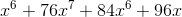Possible Answers: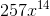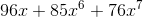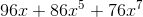The expression cannot be simplified any further

Correct answer:Explanation:

Simplify the following:We can only add variables with the same exponents.

In this case, we have two "x to the sixth" terms.

So, we treat the first x to the sixth as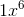Then, we add it to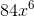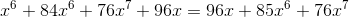### Example Question #61 : Operations

Add: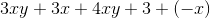Possible Answers: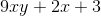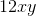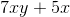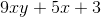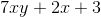Correct answer:Explanation:

In order to simplify this expression, we will need to add like terms.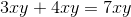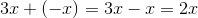There is a lone positive three.

Combine all the terms.

The answer is:1 2 3 4 5 7 Next →

### All ISEE Upper Level Math Resources